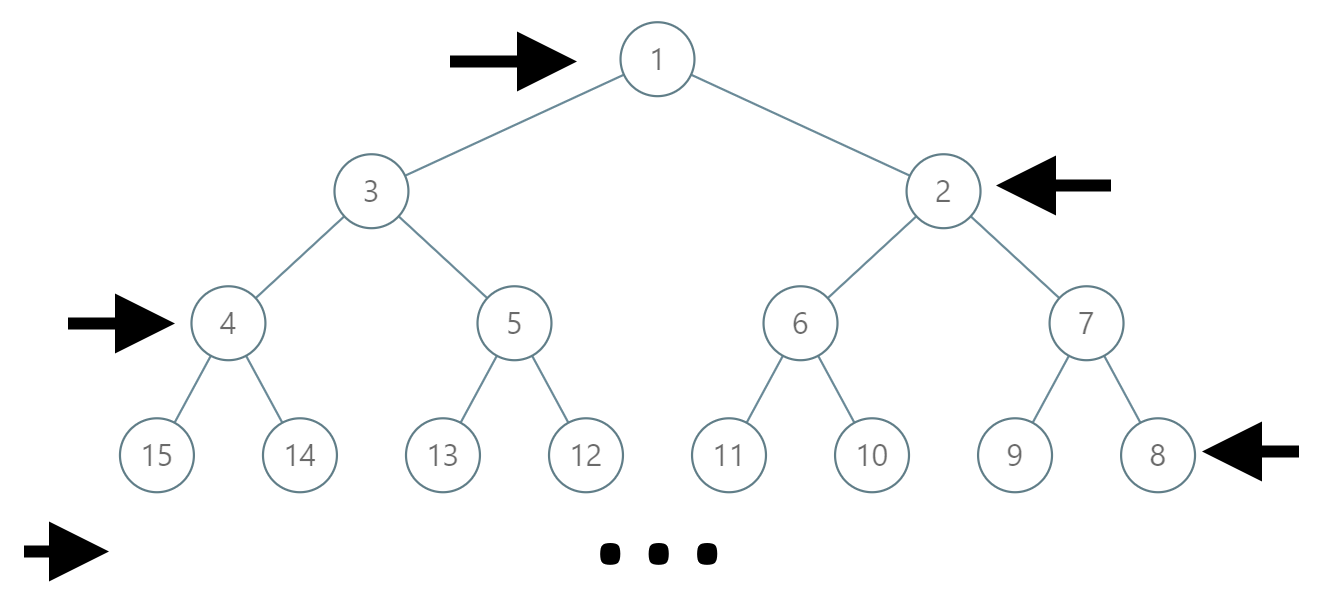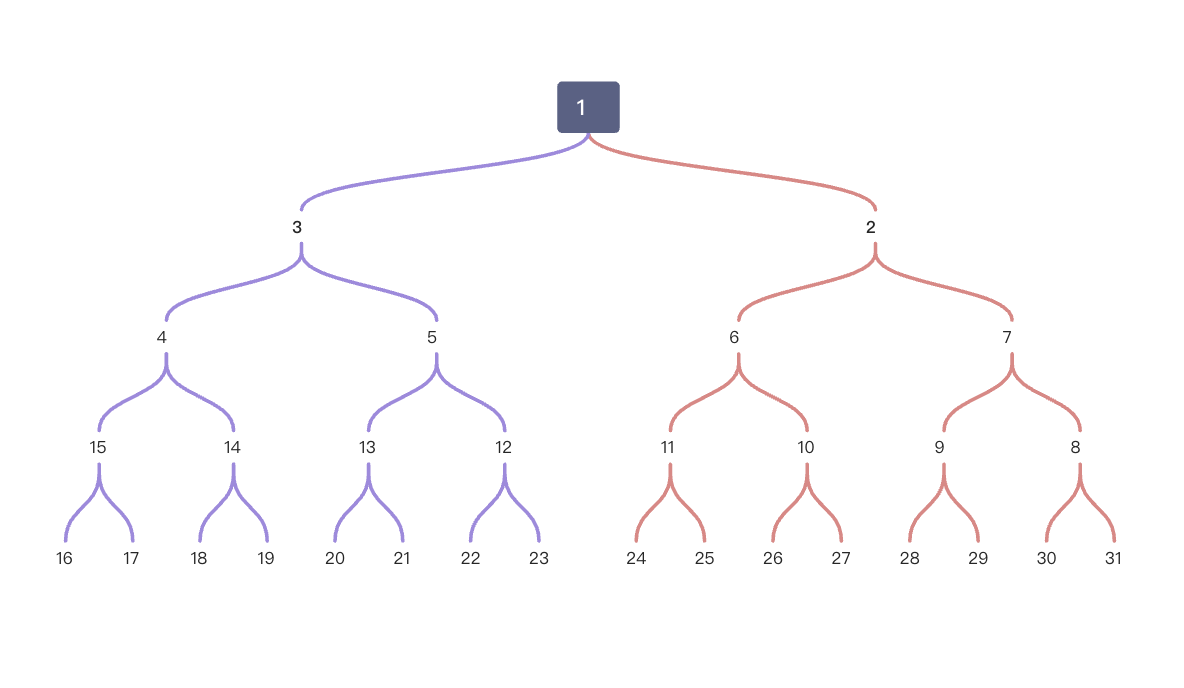## 1.1. 题目

### 1.1.1. 二叉树寻路``````输入：label = 14

``````

``````输入：label = 26

``````

• 1 <= label <= 10^6

### 1.1.2. 题解:

• 二叉树按照"之"字型排布,其实就是平常我们都是按照 层次遍历来看 二叉树,现在 一旦是偶数层,就倒转过来

### 1.1.3. 思路:

• 有个简单的思路,就是 先假设就是一个顺序的二叉树,按照层序的那种二叉树
• 然后 我们正常获取到目标节点到根节点的所有的节点,放到结果里
• 已知子节点,那么它的父节点就是 自己(偶数) / 2 或者 (自己(奇数) -1 ) / 2
• 然后看自己所在的层是 奇数层还是偶数层,

• 如果偶数层,说明自己就是对称的,但是这时候只要把 结果里的奇数层给转换了就行了
• 如果奇数层,说明自己是正常的,那么把结果里的 偶数层 给转换了就行了
• 注意 第一层和最后一层不能动,第一层是根节点,最后一层是自己
• 比如:• 假设目标节点是 8
• 按照正常的二叉树就是 `1,2,4,8`
• 但是 `8` 在偶数层,说明自己本来就是乱的,那不管,把结果里的奇数层都给换了(注意遍历 上面的结果的时候, `1,2,4,8` 里的 key+1才是层数)
• 那么就把 key = 2,层数 = key+1 = 3 给换了, 4在第三层,对应的值是 7
• 假设目标节点是31
• 按照正常的是 `1,3,7,15,31`
• 但是 `31`在 奇数层,那么把 结果里的偶数层都给换了
• 换的 方式跟上面一样

### 1.1.4. 代码:

``````func pathInZigZagTree(label int) (res []int) {
// 先得知道自己的所在的深度
level := 1
temp := label
for {
if temp>>1 == 0 {
break
}
temp = temp >> 1
level++
}

// 判断自己是在奇数层还是偶数层
var isJ bool
if level%2 != 0 {
isJ = true
}

// 先假设都是正常排序的, 从左到右的这种
// 然后放入到res里, 每个节点的父节点 等于 自己 或者自己-1 再除以2 (二叉树特性)
for {
res = append([]int{label}, res...)

if level == 1 {
break
}

if label%2 == 0 {
label = label / 2
} else {
label = (label - 1) / 2
}
level--
}

// 定义一个 交叉点,因为每层都是偶数个(除了第一层),所以分界点有两个节点 比如  4,5,6,7 分界点就是 5 和 6
// 根据找的点是奇数层还是偶数层决定应该切换哪些值(上面res 切片里的值)
var left, right int
for k, v := range res {
// 第一个值和最后一个值不修改,第一个值是1 最后一个值是要找到值本身
if k == len(res)-1 || k == 0 {
continue
}
// 如果查找的值是奇数层,那么所有偶数层就需要对称转换
// 如果查找的值是偶数层,那么所有奇数层就需要对称转换
if (isJ && (k+1)%2 == 0) || (!isJ && (k+1)%2 != 0) {
// 左对称节点是 2的层数次方 + 2的层数次方的一半
left = (1 << k) + (1<<k)/2
// 右节点等于 左节点减一
right = left - 1
// 那么对称的点就是  右节点 - 原值 + 左节点
// 如果你好奇这个公式怎么出来的,可以试试 如果你要找的原值是左边   和 是右边  看看应该是多少
res[k] = right - v + left
}
}

return res
}
``````